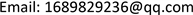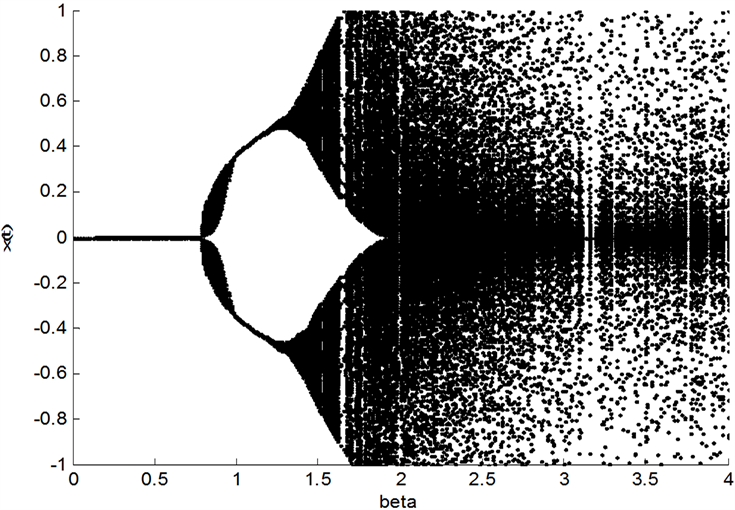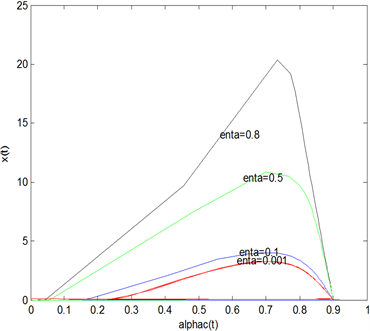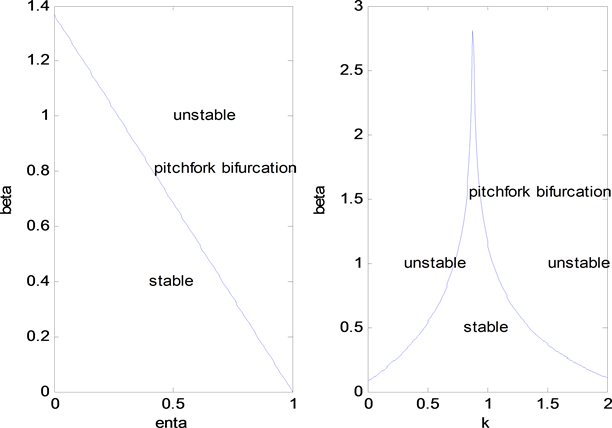﻿ 含记忆系数的资本资产定价模型 An Asset Pricing Model with Memory Coefficient

Vol.06 No.07(2017), Article ID:22553,9 pages
10.12677/AAM.2017.67108

An Asset Pricing Model with Memory Coefficient

Xuxia Li

College of Mathematics and Systems Science, Xinjiang University, Urumqi XinjiangReceived: Oct. 9th, 2017; accepted: Oct. 23rd, 2017; published: Oct. 31st, 2017ABSTRACT

This paper develops heterogeneous beliefs of the asset pricing model with the evolution fitness in the case of the two types of agents. Introducing memory coefficient, the memory parameter in deviation between the fundamentalists market fraction and chartists market fraction, we develop an asset pricing model with heterogeneous beliefs. By using the theory of difference equation, we discuss about the system of the model for local stability bifurcation analysis, and obtain the effect of main parameters on the stability of the model.

Keywords:Heterogeneous Belief, Memory Parameter, Stability1. 引言

2. 含记忆系数的动态模型

BP文献中建立的动态模型如下：

$\left\{\begin{array}{l}{x}_{t}=\frac{k}{R}\frac{1-{m}_{t-1}}{\frac{{\alpha }_{C,t}}{{\alpha }_{F}}+1+{m}_{t-1}\left(\frac{{\alpha }_{C,t}}{{\alpha }_{F}}-1\right)}{x}_{t-1},\\ {m}_{t}=\text{tanh}\left[\frac{\beta }{2{\alpha }_{F}{\sigma }^{2}}\left(R{x}_{t-1}-{x}_{t}\right)\left(R{x}_{t-1}+\frac{k{x}_{t-2}-R{x}_{t-1}}{\frac{{\alpha }_{C,t}}{{\alpha }_{F}}}\right)-\frac{\beta c}{2}\right],\\ {\alpha }_{C,t}=\left(1-\varpi \right){\overline{\alpha }}_{C}\mathrm{exp}\left[\tau \left({x}_{t-1}-R{x}_{t-2}\right)\right]+\varpi {\alpha }_{C,t-1}.\end{array}$ (1.1)

2.1. 记忆系数的引入

BP主要考虑 ${U}_{F,t}={\pi }_{F,t}$${U}_{C,t}={\pi }_{C,t}$ ，这里 ${\pi }_{h,t}$ 表示t时h类投资者的净收益， ${\pi }_{h,t}={R}_{t-1}{z}_{h,t-1}=\left({x}_{t}-R{x}_{t-1}+{\delta }_{t}\right){z}_{h,t-1}$${\delta }_{t}$ 是鞅差序列，令 ${\delta }_{t}=0$${z}_{h,t-1}$ 为t − 1时h类投资者对风险资产的需求， ${U}_{h,t}$ 为进化适应度函数。本文在此基础上引入记忆参数 $\gamma$$\eta$ ，则基本面分析者和图表分析者的进化适应度函数为：

$\left\{\begin{array}{l}{U}_{F,t}=\gamma {\pi }_{F,t}+\eta {U}_{F,t-1},\\ {U}_{C,t}=\gamma {\pi }_{C,t}+\eta {U}_{C,t-1}.\end{array}$ (1.2)

$\gamma$$\eta \in \left[0,1\right)$ 为记忆参数， $\gamma$ 衡量t − 1时h类投资者的净收益对t时的适应度函数的影响程度， $\eta$ 衡量t − 1时h类投资者的进化适应度函数对t时的适应度函数的影响程度。当 $\gamma =1,\eta =0$ 时即为BP的情形。

${m}_{t}=\mathrm{tan}h\left[\frac{\beta }{2}\left({U}_{F,t-1}-{U}_{C,t-1}\right)\right]+\delta {m}_{t-1}$(1.3)

2.2. 于是我们得到如下的非线性动态系统

$\left\{\begin{array}{l}{x}_{t}=\frac{k}{R}\frac{1-{m}_{t-1}}{\frac{{\alpha }_{C,t}}{{\alpha }_{F}}+1+{m}_{t-1}\left(\frac{{\alpha }_{C,t}}{{\alpha }_{F}}-1\right)}{x}_{t-1},\\ {m}_{t}=\text{tanh}\left[\frac{\beta }{2}\left({U}_{F,t-1}-{U}_{C,t-1}\right)\right]+\delta {m}_{t-1},\\ {\alpha }_{C,t}=\left(1-\varpi \right){\overline{\alpha }}_{C}\mathrm{exp}\left[\tau \left({x}_{t-1}-R{x}_{t-2}\right)\right]+\varpi {\alpha }_{C,t-1},\\ {U}_{F,t}=\frac{\gamma }{{\alpha }_{F}{\sigma }^{2}}R{x}_{t-1}\left(R{x}_{t-1}-{x}_{t}\right)-c+\eta {U}_{F,t-1},\\ {U}_{C,t}=\frac{\gamma }{{\alpha }_{C,t}{\sigma }^{2}}\left(k{x}_{t-2}-R{x}_{t-1}\right)\left({x}_{t}-R{x}_{t-1}\right)+\eta {U}_{C,t-1}.\end{array}$ (1.4)

$\left\{\begin{array}{l}{x}_{t}=\frac{k}{R}\frac{1-{m}_{t-1}}{\frac{{\alpha }_{C,t}}{{\alpha }_{F}}+1+{m}_{t-1}\left(\frac{{\alpha }_{C,t}}{{\alpha }_{F}}-1\right)}{x}_{t-1},\\ {y}_{t}={x}_{t-1},\\ {z}_{t}={y}_{t-1},\\ {u}_{F,t}={U}_{F,t-1},\\ {u}_{C,t}={U}_{C,t-1},\\ {m}_{t}=\mathrm{tanh}\left[\frac{\beta \gamma }{2{\alpha }_{F}{\sigma }^{2}}\left(R{y}_{t-1}-{x}_{t-1}\right)\left(R{y}_{t-1}+\frac{k{z}_{t-1}-R{y}_{t-1}}{\frac{{\alpha }_{C,t}}{{\alpha }_{F}}}\right)-\frac{\beta c}{2}+\frac{\beta \eta }{2}\left({u}_{F,t-1}-{u}_{C,t-1}\right)\right]+\delta {m}_{t-1},\\ {\alpha }_{C,t}=\left(1-\varpi \right){\overline{\alpha }}_{C}\mathrm{exp}\left[\tau \left({x}_{t-1}-R{y}_{t-1}\right)\right]+\varpi {\alpha }_{C,t-1},\\ {U}_{F,t}=\frac{\gamma }{{\alpha }_{F}{\sigma }^{2}}R{x}_{t-1}\left(R{x}_{t-1}-{x}_{t}\right)-c+\eta {U}_{F,t-1},\\ {U}_{C,t}=\frac{\gamma }{{\alpha }_{C,t}{\sigma }^{2}}\left(k{y}_{t-1}-R{x}_{t-1}\right)\left({x}_{t}-R{x}_{t-1}\right)+\eta {U}_{C,t-1}.\end{array}$ (1.5)

3. 确定性系统分析

${X}_{t}={\left({x}_{t},{y}_{t},{z}_{t},{u}_{F,t},{u}_{C,t},{m}_{t},{\alpha }_{C,t},{U}_{F,t},{U}_{C,t}\right)}^{\prime }$ ，模型的确定性系统为： $F:{R}^{9}\to {R}^{9}$ ，即 ${X}_{t}=F\left({X}_{t-1}\right)$

${F}_{1}\left({X}_{t-1}\right)=\frac{k}{R}\frac{1-{m}_{t-1}}{\frac{{F}_{7}\left({X}_{t-1}\right)}{{\alpha }_{F}}+1+{m}_{t-1}\left(\frac{{F}_{7}\left({X}_{t-1}\right)}{{\alpha }_{F}}-1\right)}{x}_{t-1}$${F}_{2}\left({X}_{t-1}\right)={x}_{t-1}$

${F}_{3}\left({X}_{t-1}\right)={y}_{t-1}$${F}_{4}\left({X}_{t-1}\right)={U}_{F,t-1}$${F}_{5}\left({X}_{t-1}\right){u}_{C,t}={U}_{C,t-1},$

${F}_{6}\left({X}_{t-1}\right)=\text{tanh}\left[\frac{\beta \gamma }{2{\alpha }_{F}{\sigma }^{2}}\left(R{y}_{t-1}-{x}_{t-1}\right)\left(R{y}_{t-1}+\frac{k{z}_{t-1}-R{y}_{t-1}}{\frac{{F}_{7}\left({X}_{t-1}\right)}{{\alpha }_{F}}}\right)-\frac{\beta c}{2}+\frac{\beta \eta }{2}\left({u}_{F,t-1}-{u}_{C,t-1}\right)\right]+\delta {m}_{t-1},$

${F}_{7}\left({X}_{t-1}\right)=\left(1-\varpi \right){\overline{\alpha }}_{C}\mathrm{exp}\left[\tau \left({x}_{t-1}-R{y}_{t-1}\right)\right]+\varpi {\alpha }_{C,t-1},$

${F}_{8}\left({X}_{t-1}\right)=\frac{\gamma }{{\alpha }_{F}{\sigma }^{2}}R{x}_{t-1}\left(R{x}_{t-1}-{F}_{1}\left({X}_{t-1}\right)\right)-c+\eta {U}_{F,t-1},$

${F}_{9}\left({X}_{t-1}\right)=\frac{\gamma }{{F}_{7}\left({X}_{t-1}\right){\sigma }^{2}}\left(k{y}_{t-1}-R{x}_{t-1}\right)\left({F}_{1}\left({X}_{t-1}\right)-R{x}_{t-1}\right)+\eta {U}_{C,t-1}$ .

${X}^{*}={\left({x}^{*},{y}^{*},{z}^{*},{u}_{F}^{*},{u}_{C}^{*},{m}^{*},{\alpha }_{C}^{*},{U}_{F}^{*},{U}_{C}^{*}\right)}^{\text{T}}$ 为其平衡解，满足 ${X}^{*}=F\left({X}^{*}\right)$ ，即为以下等式：

$\left\{\begin{array}{l}{x}^{\ast }=\frac{k}{R}\frac{1-{m}^{\ast }}{\frac{{\alpha }_{C}^{\ast }}{{\alpha }_{F}}+1+{m}^{\ast }\left(\frac{{\alpha }_{C}^{\ast }}{{\alpha }_{F}}-1\right)}{x}^{\ast },\\ {y}^{\ast }={x}^{\ast },\\ {z}^{\ast }={y}^{\ast },\\ {u}_{F}^{\ast }={U}_{F}^{\ast },\\ {u}_{C}^{\ast }={U}_{C}^{\ast },\\ {m}^{\ast }=\frac{1}{1-\delta }\text{tanh}\left[\frac{\beta \gamma }{2{\alpha }_{F}{\sigma }^{2}}\left(R-1\right)\left(R+\frac{k-R}{\frac{{\alpha }_{C}^{\ast }}{{\alpha }_{F}}}\right){\left({x}^{\ast }\right)}^{2}-\frac{\beta c}{2}+\frac{\beta \eta }{2}\left({u}_{F}^{\ast }-{u}_{C}^{\ast }\right)\right],\\ {\alpha }_{C}^{\ast }={\overline{\alpha }}_{C}\mathrm{exp}\left[\tau \left(1-R\right){x}^{\ast }\right],\\ {U}_{F}^{\ast }=\frac{1}{1-\eta }\left[\frac{\gamma }{{\alpha }_{F}{\sigma }^{2}}R\left(R-1\right){\left({x}^{\ast }\right)}^{2}-c\right],\\ {U}_{C}^{\ast }=\frac{1}{1-\eta }\frac{\gamma }{{\alpha }_{C}^{\ast }{\sigma }^{2}}\left(k-R\right)\left(1-R\right){\left({x}^{\ast }\right)}^{2}.\end{array}$ (2.1)

${m}^{eq}=\text{tanh}\left(\frac{\beta c}{2\left(\eta -1\right)}\right)$${\alpha }_{C}^{eq}={\overline{\alpha }}_{C}$${u}_{F}^{eq}={U}_{F}^{eq}=\frac{c}{\eta -1}$${u}_{C}^{eq}={U}_{C}^{eq}=0$$±{x}^{\ast }$ 为下式的解(若存在)

${m}^{\ast }=\frac{1}{1-\delta }\text{tanh}\left[\frac{\beta \gamma }{2{\alpha }_{F}{\sigma }^{2}}\left(R-1\right)\left(R+\frac{k-R}{\frac{{\alpha }_{C}^{\ast }}{{\alpha }_{F}}}\right){\left({x}^{\ast }\right)}^{2}-\frac{\beta c}{2}+\frac{\beta \eta }{2}\left({u}_{F}^{\ast }-{u}_{C}^{\ast }\right)\right]$ (2.2)

1、当 $0 时， ${X}_{0}$ 为唯一的平衡解， ${X}_{0}=\left(0,0,0,{u}_{F}^{eq},0,{m}^{eq},{\alpha }_{C}^{eq},{U}_{F}^{eq},0\right)$

2、当 $k>R\left(\frac{{\alpha }_{C}^{\ast }}{{\alpha }_{F}}+1\right),R>1$ 时，

(1) 若 $\frac{{\alpha }_{C}^{\ast }}{{\alpha }_{F}}<1$ 时，存在平衡解 ${X}_{0},{X}_{1}$${X}_{1}=\left({x}^{\ast },{x}^{\ast },{x}^{\ast },{u}_{F}^{\ast },{u}_{C}^{\ast },{m}^{\ast },{\alpha }_{C}^{\ast },{U}_{F}^{\ast },{U}_{C}^{\ast }\right)$

(2) 若 $\frac{{\alpha }_{C}^{\ast }}{{\alpha }_{F}}>1$ 时，存在平衡解 ${X}_{0},{X}_{2}$${X}_{2}=\left(-{x}^{\ast },-{x}^{\ast },-{x}^{\ast },{u}_{F}^{\ast },{u}_{C}^{\ast },{m}^{\ast },{\alpha }_{C}^{\ast },{U}_{F}^{\ast },{U}_{C}^{\ast }\right)$

3、当 $R 时，

(1) ${m}^{\ast }<{m}^{eq}$ 时， ${X}_{0}$ 是唯一的平衡解；

(2) ${m}^{\ast }>{m}^{eq}$ 时，若 $\frac{{\alpha }_{C}^{\ast }}{{\overline{\alpha }}_{C}}<1$ ，则存在平衡解 ${X}_{0},{X}_{1}$ ；若 $\frac{{\alpha }_{C}^{\ast }}{{\overline{\alpha }}_{C}}>1$ ，则存在平衡解 ${X}_{0},{X}_{2}$

1、 $0 时， $\frac{R\frac{{\alpha }_{C}^{\ast }}{{\alpha }_{F}}}{k+R\left(\frac{{\alpha }_{C}^{\ast }}{{\alpha }_{F}}-1\right)}>\frac{R\frac{{\alpha }_{C}^{\ast }}{{\alpha }_{F}}}{R+R\left(\frac{{\alpha }_{C}^{\ast }}{{\alpha }_{F}}-1\right)}=1$ ，则 ${m}^{\ast }<-1$ ，又由(2.1)第7式可知 ${\alpha }_{C}^{\ast }>0$ ，但是 ${\alpha }_{C}^{\ast }=\frac{1-{m}^{\ast }}{1+{m}^{\ast }}\left(\frac{k}{R}-1\right){\alpha }_{F}<0$ ，两者相矛盾，故此时只有平衡解 ${X}_{0}$

2、 $k>R\left(\frac{{\alpha }_{C}^{\ast }}{{\alpha }_{F}}+1\right),\text{}R>1$ 时， $0<{m}^{\ast }<1$${\alpha }_{C}^{\ast }>0$ ，因此(2.2)式存在两个平衡解 $±{x}^{\ast }$ ，有(2.1)第7式可知 ${x}^{\ast }=\frac{\mathrm{ln}\frac{{\alpha }_{C}^{\ast }}{{\overline{\alpha }}_{C}}}{\tau \left(1-R\right)}$

(1) 若 $\frac{{\alpha }_{C}^{\ast }}{{\alpha }_{F}}<1$ 时， $\mathrm{ln}\frac{{\alpha }_{C}^{\ast }}{{\overline{\alpha }}_{C}}<0$ ，所以 ${x}^{\ast }>0$ ，则存在平衡解 ${X}_{0},{X}_{1}$

(2) 若 $\frac{{\alpha }_{C}^{\ast }}{{\alpha }_{F}}>1$ 时， $\mathrm{ln}\frac{{\alpha }_{C}^{\ast }}{{\overline{\alpha }}_{C}}>0$ ，所以 ${x}^{\ast }<0$ ，则存在平衡解 ${X}_{0},{X}_{2}$

3、 $R 时， $-1<{m}^{\ast }<0$

(1) ${m}^{\ast }<{m}^{eq}$ 时，方程(2.2)无解，故只有平衡解 ${X}_{0}$

(2) ${m}^{\ast }>{m}^{eq}$ 时，方程(2.2)有解，又由(2.1)第7式得 ${x}^{\ast }=\frac{\mathrm{ln}\frac{{\alpha }_{C}^{\ast }}{{\overline{\alpha }}_{C}}}{\tau \left(1-R\right)}$ ，若 $\frac{{\alpha }_{C}^{\ast }}{{\overline{\alpha }}_{C}}<1$$\mathrm{ln}\frac{{\alpha }_{C}^{\ast }}{{\overline{\alpha }}_{C}}<0$ ，则存在平衡解 ${X}_{0},{X}_{1}$ ；若 $\frac{{\alpha }_{C}^{\ast }}{{\overline{\alpha }}_{C}}>1$$\mathrm{ln}\frac{{\alpha }_{C}^{\ast }}{{\overline{\alpha }}_{C}}>0$ ，所以 ${x}^{\ast }<0$ ，则存在平衡解 ${X}_{0},{X}_{2}$

1、 $0$\varpi <1$$\delta <1,\eta <1$ 时，基本平衡解 ${X}_{0}$ 是渐近稳定的；

2、 $k>R\left(\frac{{\alpha }_{C}^{\ast }}{{\alpha }_{F}}+1\right)$ ，或 $\varpi <1$$\delta >1$$\eta >1$ 时，基本平衡解 ${X}_{0}$ 不稳定；

3、 $R$\varpi <1$$\delta <1,\eta <1$ 时，

${m}^{\ast }<{m}^{eq}$$\frac{{\alpha }_{C}^{\ast }}{{\overline{\alpha }}_{C}}<1$ 时，基本平衡解 ${X}_{0}$ 是渐近稳定的；

4、当 $\varpi <1,\delta <1,\eta <1$$\frac{k}{R}\frac{1-{m}^{eq}}{\frac{{\overline{\alpha }}_{C}}{{\alpha }_{F}}+1+{m}^{eq}\left(\frac{{\overline{\alpha }}_{C}}{{\alpha }_{F}}-1\right)}=1$ 时，出现音叉分支，当 $0<\beta <{\beta }^{\ast }$ 时，基本平衡解 ${X}_{0}$ 稳定，当 $\beta >{\beta }^{\ast }$ 时，基本平衡解 ${X}_{0}$ 不稳定， ${\beta }^{\ast }=\frac{\eta -1}{c}\mathrm{ln}\left[\frac{{\alpha }_{F}}{{\overline{\alpha }}_{C}}\left(1-\frac{k}{R}-\frac{{\overline{\alpha }}_{C}}{{\alpha }_{F}}\right)\right]$

$\frac{\partial {F}_{1}}{\partial x}=\frac{k}{R}\frac{1-{m}^{eq}}{\frac{{\overline{\alpha }}_{C}}{{\alpha }_{F}}+1+{m}^{eq}\left(\frac{{\overline{\alpha }}_{C}}{{\alpha }_{F}}-1\right)}=A$ , $\frac{\partial {F}_{1}}{\partial X}=0$ , $\frac{\partial {F}_{2}}{\partial x}=1$ , $\frac{\partial {F}_{2}}{\partial X}=0$ , $\frac{\partial {F}_{3}}{\partial y}=1$ , $\frac{\partial {F}_{3}}{\partial X}=0$ , $\frac{\partial {F}_{4}}{\partial {U}_{F}}=1$ , $\frac{\partial {F}_{4}}{\partial X}=0$ , $\frac{\partial {F}_{5}}{\partial {U}_{C}}=1$ , $\frac{\partial {F}_{5}}{\partial X}=0$ , $\frac{\partial {F}_{6}}{\partial {u}_{F}}=\frac{\beta \eta }{2}\text{tan h ′}\left(\theta \right)=B$ , $\frac{\partial {F}_{6}}{\partial m}=\delta$ , $\frac{\partial {F}_{6}}{\partial {u}_{C}}=-\frac{\beta \eta }{2}\text{tan h ′}\left(\theta \right)=C$ , $\frac{\partial {F}_{6}}{\partial X}=0$ , $\frac{\partial {F}_{7}}{\partial x}=\tau \left(1-\varpi \right){\overline{\alpha }}_{C}=D$ , $\frac{\partial {F}_{7}}{\partial {\alpha }_{C}}=\varpi$ , $\frac{\partial {F}_{7}}{\partial y}=-\tau R\left(1-\varpi \right){\overline{\alpha }}_{C}=E$ , $\frac{\partial {F}_{7}}{\partial X}=0$ , $\frac{\partial {F}_{8}}{\partial {U}_{F}}=\eta$ , $\frac{\partial {F}_{8}}{\partial X}=0$ , $\frac{\partial {F}_{9}}{\partial {U}_{C}}=\eta$ , $\frac{\partial {F}_{9}}{\partial X}=0$ .

$J=\left[\begin{array}{ccccccccc}A& 0& 0& 0& 0& 0& 0& 0& 0\\ 1& 0& 0& 0& 0& 0& 0& 0& 0\\ 0& 1& 0& 0& 0& 0& 0& 0& 0\\ 0& 0& 0& 0& 0& 0& 0& 1& 0\\ 0& 0& 0& 0& 0& 0& 0& 0& 1\\ 0& 0& 0& B& C& \delta & 0& 0& 0\\ D& E& 0& 0& 0& 0& \varpi & 0& 0\\ 0& 0& 0& 0& 0& 0& 0& \eta & 0\\ 0& 0& 0& 0& 0& 0& 0& 0& \eta \end{array}\right]$

$\begin{array}{c}|\lambda E-J|=|0\begin{array}{ccccccccc}\lambda -A& 0& 0& 0& 0& 0& 0& 0& 0\\ -1& \lambda & 0& 0& 0& 0& 0& 0& 0\\ 0& -1& \lambda & 0& 0& 0& 0& 0& 0\\ 0& 0& 0& \lambda & 0& 0& 0& 0& 0\\ 0& 0& 0& 0& \lambda & 0& 0& 0& 0\\ 0& 0& 0& -B& -C& \lambda -\delta & 0& 0& 0\\ -D& -E& 0& 0& 0& 0& \lambda -\varpi & 0& 0\\ 0& 0& 0& 0& 0& 0& 0& \lambda -\eta & 0\\ 0& 0& 0& 0& 0& 0& 0& 0& \lambda -\eta \end{array}|\\ =\left(\lambda -A\right)\left(\lambda -\varpi \right)\left(\lambda -\delta \right){\left(}^{\lambda }{\lambda }^{4}=0,\end{array}$

${\lambda }_{1}=A=\frac{k}{R}\frac{1-{m}^{eq}}{\frac{{\overline{\alpha }}_{C}}{{\alpha }_{F}}+1+{m}^{eq}\left(\frac{{\overline{\alpha }}_{C}}{{\alpha }_{F}}-1\right)}$${\lambda }_{2}=\varpi$${\lambda }_{3}=\delta$${\lambda }_{4,5}=\eta$${\lambda }_{6,7,8,9}=0$

1、因为 $\frac{1-{m}^{eq}}{\frac{{\overline{\alpha }}_{C}}{{\alpha }_{F}}+1+{m}^{eq}\left(\frac{{\overline{\alpha }}_{C}}{{\alpha }_{F}}-1\right)}=\frac{1-{m}^{eq}}{\frac{{\overline{\alpha }}_{C}}{{\alpha }_{F}}\left({m}^{eq}+1\right)+1-{m}^{eq}}<1$ ，所以当 $0 时， ${\lambda }_{1}<1$

$\varpi <1$ 时， ${\lambda }_{2}<1$$\delta <1$ 时， ${\lambda }_{3}<1$$\eta <1$ 时， ${\lambda }_{4,5}<1$ ，故基本平衡解 ${X}_{0}$ 是渐近稳定的；

2、 $k>R\left(\frac{{\alpha }_{C}^{\ast }}{{\alpha }_{F}}+1\right)$ 时， ${\lambda }_{1}>1$$\varpi >1$ 时， ${\lambda }_{2}>1$$\delta >1$ 时， ${\lambda }_{3}>1$$\eta >1$ 时， ${\lambda }_{4,5}>1$

3、 $R${m}^{\ast }<{m}^{eq}$ 时方程(2.2)无解，若 $\frac{{\alpha }_{C}^{\ast }}{{\overline{\alpha }}_{C}}<1$ ，则 ${\lambda }_{1}<1$ ，且 $\varpi <1$$\delta <1$$\eta <1$ 时， ${\lambda }_{2}<1$${\lambda }_{3}<1$${\lambda }_{4,5}<1$ ，所以基本平衡解 ${X}_{0}$ 是渐近稳定的；

4、 $\varpi <1,\delta <1,\eta <1$${\lambda }_{1}=1$ 时，此时特征根都为非负实数，有且仅有可能出现pitchfork分支，即 $\frac{k}{R}\frac{1-{m}^{eq}}{\frac{{\overline{\alpha }}_{C}}{{\alpha }_{F}}+1+{m}^{eq}\left(\frac{{\overline{\alpha }}_{C}}{{\alpha }_{F}}-1\right)}=1$ ，解得 ${\beta }^{\ast }=\frac{\eta -1}{c}\mathrm{ln}\left(\frac{\left(2-\delta \right)\left(\frac{k}{R}-1\right)+\delta \frac{{\overline{\alpha }}_{C}}{{\alpha }_{F}}}{\delta \left(\frac{k}{R}-1\right)+\left(2-\delta \right)\frac{{\overline{\alpha }}_{C}}{{\alpha }_{F}}}\right)$ ，故当 $0<\beta <{\beta }^{\ast }$ 时，基本平衡解 ${X}_{0}$ 稳定，当 $\beta >{\beta }^{\ast }$ 时，基本平衡解 ${X}_{0}$ 不稳定。

$R=1.1$ , ${\alpha }_{F}=1$ , $\varpi =0.1$ , $\tau =1$ , ${\overline{\alpha }}_{C}=0.9$ , ${\sigma }^{2}=1$ , $c=1$ , $k=0.5$ , $\eta =0.5$ , $\gamma =0.5$ , $\delta =0.2$ .Figure 1. The sensitivity of β to deterministic systemsFigure 2. $\left({\alpha }_{C,t},{x}_{t}\right)$ plane by the change of γ and ηFigure 3. The effect of n and k on β

4. 总结

An Asset Pricing Model with Memory Coefficient[J]. 应用数学进展, 2017, 06(07): 896-904. http://dx.doi.org/10.12677/AAM.2017.67108

1. 1. Friedman, M. (1953) The Case of Flexible Exchange Rates. In: Essays in Positive Economics, University of Chicago Press, Chica-go.

2. 2. LeBaron, B. (2001) Evolution and Time Horizons in an Agent-Based Stock Market. Macroeconomic Dynamics, 5, 225-254. https://doi.org/10.1017/S1365100501019058

3. 3. LeBaron, B. (2002) Short-Memory Traders and Their Impact on Group Learning in Financial Markets. Proceedings of the National Academy of Sciences of the United States of America, 99, 7201-7206. https://doi.org/10.1073/pnas.072079699

4. 4. Hommes, C., Kiseleva, T., et al. (2012) Is More Memory in Evolutionary Selection (De)Stabilizing? Macroeconomic Dynamics, 16, 335-357. https://doi.org/10.1017/S136510051000060X

5. 5. Beum-Jo, P. (2014) Heterogeneous Risk Aversion and Dynamics of Asset Prices among Bounded Rational Agents. Journal of Banking & Finance, 43, 150-159.